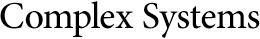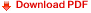## Comparison of Dynamic Diffusion with an Explicit Difference Scheme for the Schrödinger EquationV. Kukushkin
Y. I. Ozhigov
Moscow State University of M. V. Lomonosov
Institute of Physics and Technology RAS

#### Abstract

We represent the method of dynamic diffusion for the approximate solution of Schrödinger's equation with decoherence and describe a swarm simulation as a proof of concept. Decoherence occurs as the divergence of the exact solution for the dynamics of the diffusion swarm, which arises when the total number of real particles grows. The method of dynamic diffusion cannot be reduced to the solution of differential equations, in contrast to Bohm's quantum hydrodynamics; hence, direct computer simulation is the mandatory next step. We compare the dynamic diffusion with the explicit finite differences scheme for the Schrödinger equation for some standard one-dimension cases.

https://doi.org/10.25088/ComplexSystems.22.4.423